GCSE Maths Algebra Maths Formulas

Rearranging Formulae

# Rearranging Formulae

Here we will learn about rearranging formulae, including changing the subject of a formula, as well as formulae for perimeter, area, volume, Pythagoras theorem and other areas of mathematics.

There are also rearranging formulae worksheets based on Edexcel, AQA and OCR exam questions, along with further guidance on where to go next if you’re still stuck.

## What is rearranging formulae?

Rearranging formulae is a way of changing the subject of a formula. This can help us determine a missing value when we know other values within a formula.

Change subject of a formula

To change the subject of a formula is to rewrite the formula so a single unknown variable is equal to the rest of it.

We can use this technique to rearrange equations and solve equations.

Step-by-step guide: Rearranging equations

Step-by-step guide: Solving equations

To rearrange formulae, we need to use inverse operations.

Below is a table of operations with their mathematical inverse.

• The formulae may contain brackets.

In order to remove brackets, you need to be able to expand brackets (multiply each term within the bracket by the value next to the bracket).

For example,

The perimeter of a rectangle can be expressed as the formula P=2(l+w) where P is the subject of the formula, and l and w are the length and width of the rectangle.

In order to find the width of the rectangle given the perimeter and length, we need to rearrange the formula to make w the subject.

To do this we first need to expand the bracket to get P=2l+2w, then we can use inverse operations to subtract 2l from both sides of the equals sign and divide everything by 2.

Step-by-step guide: Expanding brackets

• The formulae may contain fractions

To remove a fraction, we must multiply the formula throughout by the denominator of the fraction.

For example,

Rearrange the formula to make x the subject.

\frac{5}{x}=2y

We need to multiply both sides of the equals sign by the denominator of the fraction x, and then use inverse operations to divide everything by 2y.

Step-by-step guide: Equations with fractions

### What is rearranging formulae?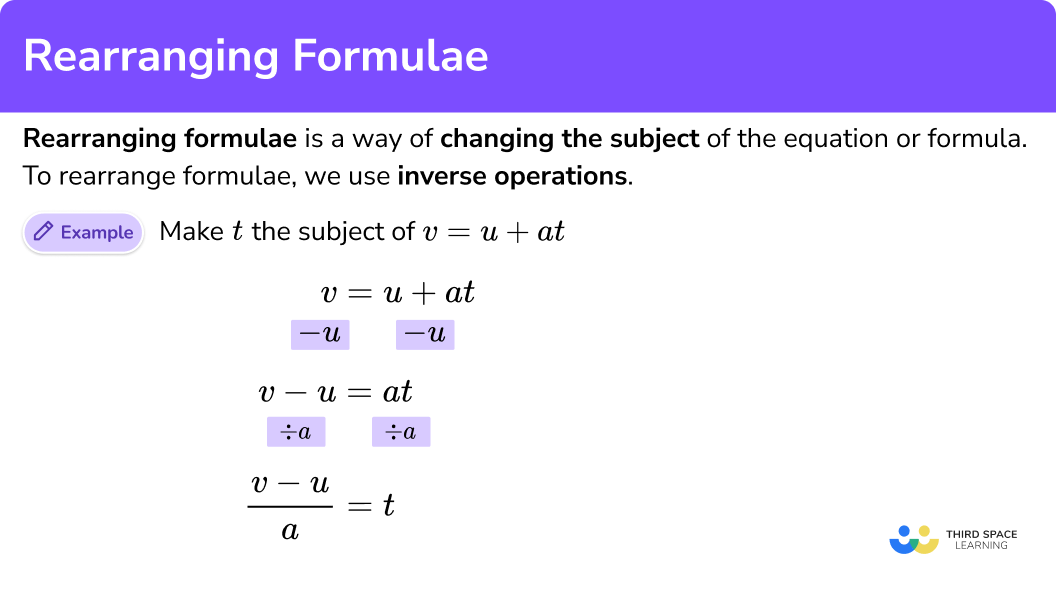## How to rearrange formulae

In order to rearrange formulae:

1. Use inverse operations to move a number, term, or variable.
2. Repeat this process until the remaining variable is the subject.

### Explain how to rearrange formulae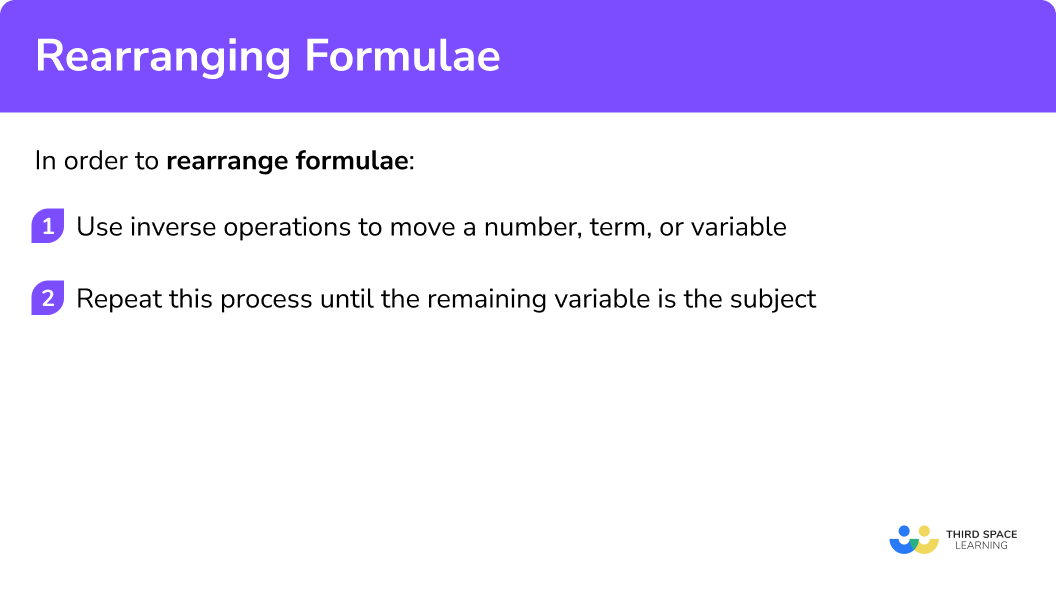## Rearranging formulae examples

### Example 1: area of a rectangle

Make h the subject of the formula A=wh.

1. Use inverse operations to move a number, term, or variable.

To make h the subject of the formula, we need to remove w from the right-hand side of the equation.

As wh is the same as w\times{h} and multiplication is commutative, we can state that A=h\times{w}.

As h is being multiplied by w, to remove w from the right hand side of the equation, we need to divide both sides of the equation by w.

2Repeat this process until the remaining variable is the subject.

The remaining variable h is now the subject, however it is standard practice to write this variable first and so we have the final solution,

h=\frac{A}{w}.

Why is this useful?

This formula would calculate the height of a rectangle (h) given the area (A) and the width of the rectangle (w).

### Example 2: equation of a straight line

Make x the subject of the formula for the equation of a straight line y=mx+c.

As the variable c is being added to the term mx, we can subtract c from both sides of the equation to get,

As mx is the same as m \times x, we need to divide the right hand side of the equation by m to leave x. If we divide the right hand side of the equation by m, we must do the same to the left-hand side of the equation and so we have,

This makes x the subject of the equation, however we need to write the subject first. Changing the sides of the equation, we have the solution

x=\frac{y-c}{m}.

Why is this useful?

This formula would calculate the coordinate x, given the coordinate y, the y -intercept (c) and the gradient of the line (m).

### Example 3: Pythagoras theorem

Make a the subject of Pythagoras theorem, a^{2}+b^{2}=c^{2}.

As b^2 is being added to a^{2}, we can remove b^2 from the left hand side of the equation by subtracting it.

We currently have a^{2} as the subject of the equation. To get a to be the subject, we need to square root both sides of the equation to get,

This gives us the final solution,

a=\sqrt{c^{2}-b^{2}}.

Why is this useful?

This formula would calculate the length of a shorter side of a right angled triangle, a, given the hypotenuse, c, and the other side of the triangle, b.

### Example 4: volume of a sphere

Make r the subject of the formula V=\frac{4}{3}\pi{r}^{3}.

As \frac{4}{3}\pi{r^{3}} is the same as 4\pi{r^{3}} being divided by 3, we need to multiply both sides by 3 to remove the fraction.

As r^3 is being multiplied by 4 \pi we can divide both sides of the equation by 4 \pi to get,

Calculating the cube root of each side of the equation will make r the subject of the formula.

We therefore have the solution,

r=\sqrt{\frac{3V}{4\pi}}.

Why is this useful?

This formula would calculate the radius of the sphere r, given the volume of the sphere, V.

### Example 5: surface area of a cone

Make l the subject of the formula A=\pi{r}^{2}+\pi{rl}.

As we want l to be the subject of the formula, we can remove the term \pi r^2 from the right hand side of the equation by subtracting it from both sides of the equation (keeping it balanced).

As l is being multiplied by \pi r, if we divide both sides of the equation by \pi r, we will only have l remaining on one side of the equation, which will mean that l is the subject of the equation.

The solution is therefore

l=\frac{A-\pi{r}^{2}}{\pi{r}}.

Why is this useful?

This formula would calculate the slant length l of a cone, given the radius of the base of the cone, r, and the total surface area of the cone, A.

### Example 6: compound interest formula

Make r the subject of the formula V=P(1+r)^{t}.

As (1+r)^t is being multiplied by P, we can divide both sides of the equation by P to get,

As (1+r) is being raised to the power of t, we need to find the t^{th} root of both sides of the equation.

This leaves us with r+1 on the right hand side of the equation and so we can simply subtract 1 from both sides of the equation to make r the subject.

The solution is therefore

r=\sqrt[t]{\frac{V}{P}}-1.

Why is this useful?

This formula would calculate the percentage change, r, given the initial value, P, the new value, V, and the frequency of times the initial value has been changed, t.

### Common misconceptions

• Incorrect inverse operation

The inverse operation used is incorrect. For example, let M=DV. Here, the formula is rearranged to get M-V=D and so the value of V has been subtracted, rather than divided. The correct answer should be \frac{M}{V}=D.

• Moving letters

Letters are simply moved from one side of the equals sign to the other. For example, A=lw means that Al=w which is incorrect. Instead we need to use the inverse operation to multiplying by l, which is to divide both sides by l to get w=\frac{A}{l}.

• Not multiplying or dividing throughout

When given the equation y=mx+c, if we divided both sides of the equation by m we should get \frac{y}{m}=x+\frac{c}{m} whereas students would incorrectly write \frac{y}{m}=x+c. When multiplying and dividing by something, every term on each side of the equals sign must be multiplied or divided by it.

• Expanding brackets

When expanding brackets, all terms within the bracket must be multiplied by the value on the outside of the bracket. For example, expanding the brackets of 2(x+3), we get 2x+6 as both of the terms inside the bracket have been multiplied by 2.

• The power disappears

When we have a variable raised to a power, we must use inverse operations to remove that power. For example, if we have x^{4} we need to calculate the 4th root of the variable to get x. We cannot disregard the power, for example x^{4}=16 so x=16 is incorrect. The correct answer is x=2.

• Factorising to get one variable

The surface area of a cone is written as A=\pi{r^2}+\pi{rl}. If we wanted to make \pi the subject, students write \pi=\frac{A-\pi{r^2}}{rl} which has \pi occurring on both sides of the equation and therefore we need to know the value of \pi to calculate the value of \pi, which we cannot do.

Instead, we need to factorise the right hand side of the equation in terms of \pi so we get A=\pi(r^{2}+rl). Dividing both sides by r^{2}+rl we get \pi=\frac{A}{r^{2}+rl} which calculates the value of \pi given the total surface area of a cone (A), the radius of the base (r), and the slant length (l).

### Practice rearranging formulae questions

1. Make S the subject of the formula D=ST.

S=DT\frac{T}{D}=SS=\frac{D}{T}D-T=S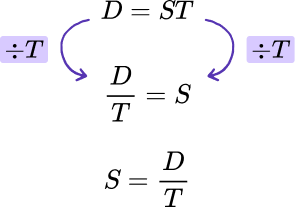2. Make a the subject of the formula v=u+at.

a=\frac{v-u}{t}a=v-u-tu=v-ata=\frac{u-v}{t}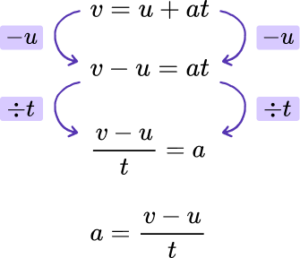3. Make b the subject of Pythagoras theorem a^{2}+b^{2}=c^{2}.

b=c-aa=\frac{c^{2}-b^{2}}{2}b=\sqrt{c^{2}+a^{2}}b=\sqrt{c^{2}-a^{2}}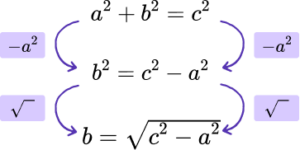4. Make b the subject of the formula V=\frac{b^{2}h}{2}.

b=2V\div{h}b=\sqrt{\frac{2V}{h}}b=\frac{\sqrt{V}h}{2}b=\sqrt{2Vh}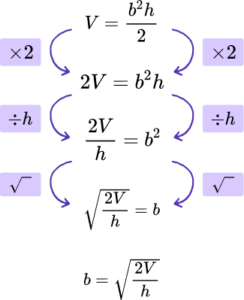5. Make a the subject of s=ut+ \frac{1}{2}at^{2}.

a=\frac{2s-ut}{t^{2}}a=\frac{2(s-ut)}{t^{2}}a=\frac{sut}{2t^{2}}a=2t^{2}(s-ut)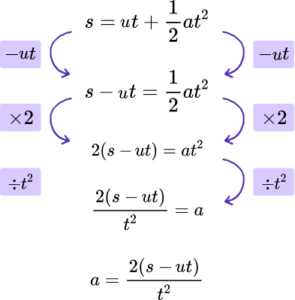6. Make d the subject of r=\sqrt{\frac{Ad}{C}}.

d=\frac{r^{2}C}{A}d=\frac{(rc)^{2}}{A}d=Ar^{2}Cd=AC\sqrt{r}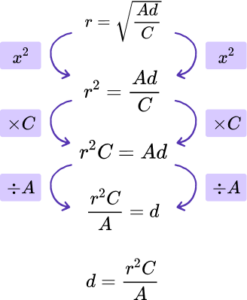### Rearranging formulae GCSE questions

1. Make b the subject of the formula for the area of a trapezium.

A = \frac{h(a+b)}{2}

(3 marks)

Multiplied both sides by 2 to eliminate the fraction, 2A=(a+b) \times h .

(1)

Divided both sides by h, \ \frac{2A}{h}=a+b .

(1)

Subtracts a, and get b=\frac{2A}{h}-a .

(1)

2. (a) Make h the subject of the formula \sin(\theta)=\frac{x}{h}.

(b) Make \theta the subject of \cos(\theta)=\frac{A}{H}.

(3 marks)

(a)

Multiply both sides by h, \ h \times \sin(\theta)=x .

(1)

Divide both sides by \sin(\theta), \ h= \frac{x}{\sin(\theta)} .

(1)

(b)

Use \cos^{-1} as the inverse operation for \cos, \theta =\cos^{-1}(\frac{A}{H}) .

(1)

3. (a) Make C the subject of A=\frac{1}{2}ab \sin(C).

(b) Make \theta the subject of the cosine rule,

c^{2}=a^{2}+b^{2}-2ab \cos(\theta).

(7 marks)

(a)

Multiply both sides by 2 to eliminate the fraction, 2A=ab \sin(C) .

(1)

Divide both sides by ab, \ \frac{2A}{ab}= \sin(C) .

(1)

Use \sin^{-1} as the inverse operation for \sin, \ C =\sin^{-1}(\frac{2A}{ab}) .

(1)

(b)

Add 2ab \cos(\theta) to both sides, c^{2}+2ab \cos(\theta)=a^{2}+b^{2} .

(1)

Subtract c^2 from both sides, 2ab \cos(\theta)=a^{2}+b^{2}-c^{2} .

(1)

Divide both sides by 2ab, \ \cos(\theta)=\frac{a^{2}+b^{2}-c^{2}}{2ab} .

(1)

Use \cos^{-1} as the inverse operation for \cos, \ \theta =\cos^{-1}(\frac{a^{2}+b^{2}-c^{2}}{2ab}) .

(1)

## Learning checklist

You have now learned how to:

• Understand and use inverse operations
• Understand and use standard mathematical formulae
• Rearrange formulae to change the subject

## Still stuck?

Prepare your KS4 students for maths GCSEs success with Third Space Learning. Weekly online one to one GCSE maths revision lessons delivered by expert maths tutors.

Find out more about our GCSE maths revision programme.